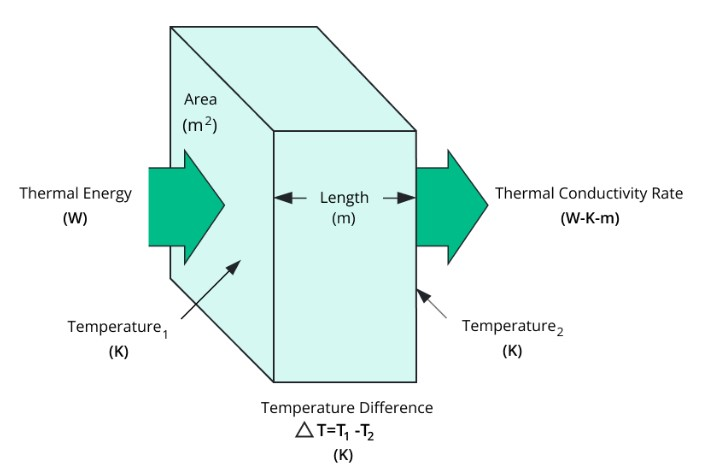# Thermal Conductivity Calculator

Select the unknown term, enter the required values, and hit the calculate button

calorie/degree-cm-s
cm

Give Us Feedback

## Thermal Conductivity Calculator

Thermal Conductivity Calculator is used to find the thermal conductivity of solid material using the physical properties and dimensions of the material.

To determine the material's thermal conductivity using the thickness of the material, the temperature differential between its ends, and the area where heat flows were taken into consideration.

## What is the Thermal Conductivity?

It is the ability of the material to conduct heat and is defined as the capacity of heat which flows under the area of material in unit time where the temperature difference between the two ends of the material.

### Thermal Conductivity Formula

Thermal Conductivity's general formula is represented by the following.

Thermal Conductivity = (Heat × Length of material) / (Area × Temperature Difference)In mathematical terms is also expressed as.

k = (Q × L) / (A × ΔT)

Where, k = thermal conductivity

• Q = Heating rate for specific material
• L = Length of the used material
• A = surface area of material under observation
• ΔT = Temperature variation within the specified material from end to end

## How to find the unknown term of thermal conductivity formula?

Example 1:

If the 0.29 m long object one end is dipped in steam and the other end is merged in ice then find the thermal conductivity. Given that amount of heat is 1200 calories, the change in temperature is 600 kelvin, and the cross-section of an object is 5 × 10-2m2.

Solution:

Step 1:

Write the data carefully from the question.

k = thermal conductivity =?,                           Q = heat rate of a given object = 1200

L = Length of the used object = 0.29 m,         A = surface area of an object = 5 × 10-2m2

ΔT = temperature difference = 600 kelvin

Step 2:

Written the Formula of Thermal Conductivity.

k = (Q × L) / (A × ΔT)

Step 3:

Put the values in the above formula and simplify.

Q = 1200, L = 0.29 m, A = 5 × 10-2m2, ΔT = 600 kelvin

k = (Q × L) / (A × ΔT)

k = (1200 × 0.29) / (5 × 10-2 × 600)

k = (348) / (30)

k = 11.6

Example 2:

Evaluate the thermal conductivity of the object if one end of an object is 5m long placed in air and the other end is placed in fire. Given that amount of heat is 1600 calories, the change in temperature is 200 kelvin, and the cross-section of an object is 60 × 10-3m2.

Solution:

Step 1:

Write the data carefully from the question.

k = thermal conductivity =?,                           Q = heat rate of a given object = 1600

L = Length of the used object = 5m,               A = surface area of an object = 60 × 10-3m2

ΔT = temperature difference = 200 kelvin

Step 2:

Written the Formula of Thermal Conductivity.

k = (Q × L) / (A × ΔT)

Step 3:

Put the values in the above formula and simplify.

Q = 1600, L = 5 m, A = 60 × 10-3m2, ΔT = 200 kelvin

k = (Q × L) / (A × ΔT)

k = (1600 × 5) / (60 × 10-3 × 200)

k = (8000) / (12)

k = 666.67

### Math Tools